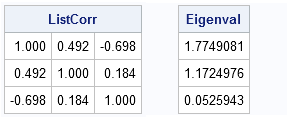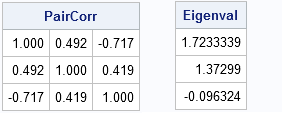It is "well known" that the pairwise deletion of missing values and the resulting computation of correlations can lead to problems in statistical computing. I have previously written about this phenomenon in my article "When is a correlation matrix not a correlation matrix."

Specifically, consider the symmetric array whose elements are pairwise correlations between variables. This array is not always a valid correlation matrix because the array can lack a property called "positive definiteness." However, when I tried to find a simple example that demonstrated this result, all I could find were statements about how well known the result is! Consequently, I decided to create a simple example.

The following data matrix, which is input by using PROC IML, contains six observations for three variables. The first observation contains a missing value for the X1 variable. The second observation contains a missing value for the X2 variable.

```proc iml; X = {. 3 2, 8 . 2, 1 5 8, 1 3 5, 2 4 3, 4 5 3 };```

Listwise deletion is the operation used by regression procedures to deal with missing values. During listwise deletion, an observation that contains a missing value in any variable is discarded; no portion of that observation is used when building "cross product" matrices such as the covariance or correlation matrix. For our example, listwise deletion means that the correlation matrix is formed by using rows 3–6, as follows:

```/* Listwise deletion matrix: 1 5 8, 1 3 5, 2 4 3, 4 5 3 */ ListCorr = corr(X, "Pearson", "listwise"); Eigenval = eigval(ListCorr); print ListCorr[format=6.3], Eigenval;```Notice that all eigenvalues of the correlation matrix are positive. This is a mathematical fact: a valid correlation matrix has nonnegative eigenvalues.

What happens if you form the matrix that consists of pairwise correlations? That is, form the array C such that C[i,j] is the correlation between the ith and the jth columns of X. The missing values for each pair of variables are deleted based on whether either variable contains a missing value.

Under this pairwise-deletion scheme, each element of C is computed by using different observations:

• The element C[1,2] is computed by using observations 3–6 because the first observation has a missing value for X1 and the second observation has a missing value for X2.
• The element C[1,3] is computed by using observations 2–6 because the first observation is missing for X1.
• The element C[2,3] is computed by using observations 1 and 3–6 because the second observation is missing for X2.

The following SAS/IML statements compute the array of pairwise correlations:

```/* vectors used for pairwise correlation R12 R13 R23 3 2 8 2 1 5 1 8 5 8 1 3 1 5 3 5 2 4 2 3 4 3 4 5 4 3 5 3 */ PairCorr = corr(X, "Pearson", "pairwise"); Eigenval = eigval(PairCorr); print PairCorr[format=6.3], Eigenval;```For the matrix of pairwise correlations, one eigenvalue is negative. This indicates that the matrix is not a valid correlation matrix. There is no multivariate distribution for which this matrix represents the correlation between variables!

By default PROC CORR computes pairwise correlations. If your variables contain missing values, the resulting matrix might not be a true correlation matrix. If you intend to use the PROC CORR output for simulation or as input for a regression or multivariate analysis, be sure to specify the NOMISS option on the PROC CORR statement! This option performs listwise deletion of observations with missing values and always results in valid correlation matrix.

Share